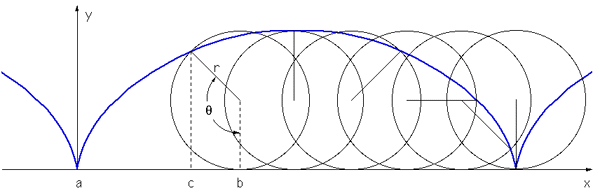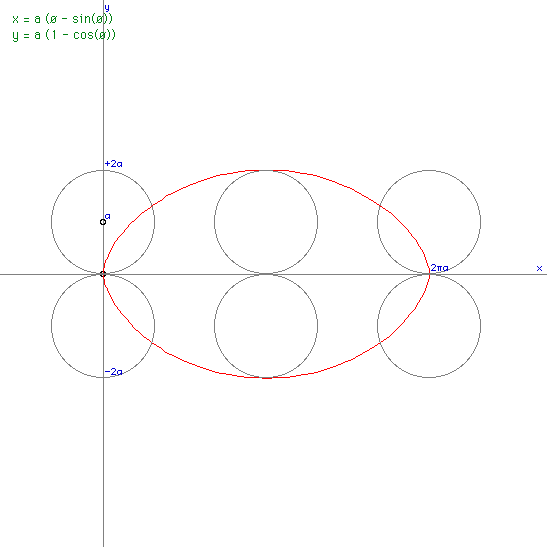# Cycloid Curve

Written by Paul Bourke
June 1997

Everyone has witnessed a rolling wheel or tyre, have you ever stopped to wonder what curve is traced out by a point on the rim of the wheel? Such a curve is called a cycloid. Consider the circle below of radius "r".The equation for the curve traced out is given by:

 x = r (theta - sin(theta)) y = r (1 - cos(theta))

This can is derived by noting that the distance between a and b is the arc (r theta). So x = distance a -> b, minus distance c -> b. This second term is r sin(theta). The height y = r - r cos(theta).